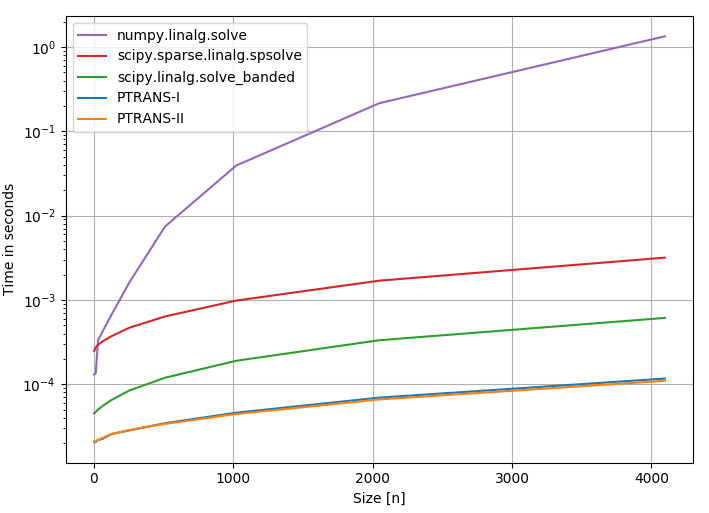# pentapy Quickstartpentapy is a toolbox to deal with pentadiagonal matrices in Python and solve the corresponding linear equation systems.

## Installation

The package can be installed via pip. On Windows you can install WinPython to get Python and pip running.

```pip install pentapy
```

There are pre-built wheels for Linux, MacOS and Windows for most Python versions.

To get the scipy solvers running, you have to install scipy or you can use the extra argument:

```pip install pentapy[all]
```

Instead of “all” you can also typ “scipy” or “umfpack”.

## Examples

### Solving a pentadiagonal linear equation system

This is an example of how to solve a LES with a pentadiagonal matrix.

```import numpy as np
import pentapy as pp

size = 1000
# create a flattened pentadiagonal matrix
M_flat = (np.random.random((5, size)) - 0.5) * 1e-5
V = np.random.random(size) * 1e5
# solve the LES with M_flat as row-wise flattened matrix
X = pp.solve(M_flat, V, is_flat=True)

# create the corresponding matrix for checking
M = pp.create_full(M_flat, col_wise=False)
# calculate the error
print(np.max(np.abs(np.dot(M, X) - V)))
```

This should give something like:

```4.257890395820141e-08
```

### Performance

In the following, a couple of solvers for pentadiagonal systems are compared:

• Solver 1: Standard linear algebra solver of Numpy `np.linalg.solve` (link)

• Solver 2: `scipy.sparse.linalg.spsolve` (link)

• Solver 3: Scipy banded solver [`scipy.linalg.solve_banded`](scipy.github.io/devdocs/generated/scipy.linalg.solve_banded.html)

• Solver 4: pentapy.solve with `solver=1`

• Solver 5: pentapy.solve with `solver=2`The performance plot was created with `perfplot` (link).